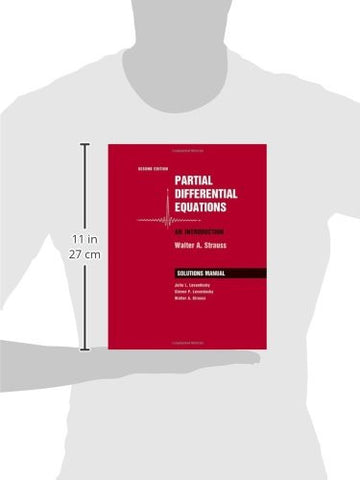# Student Solutions Manual to accompany Partial Differential Equations: An Introduction, 2nd Edition

• ISBN-13: 9780470260715
• \$114.16

Only 3 left!

Our understanding of the fundamental processes of the natural world is based to a large extent on partial differential equations (PDEs). The second edition of Partial Differential Equations provides an introduction to the basic properties of PDEs and the ideas and techniques that have proven useful in analyzing them. It provides the student a broad perspective on the subject, illustrates the incredibly rich variety of phenomena encompassed by it, and imparts a working knowledge of the most important techniques of analysis of the solutions of the equations. In this book mathematical jargon is minimized. Our focus is on the three most classical PDEs, the wave, heat and Lapace equations. Advanced concepts are introduced frequently but with the least possible technicalities. The book is flexibly designed for juniors, seniors or beginning graduate students in science, engineering or mathematics.